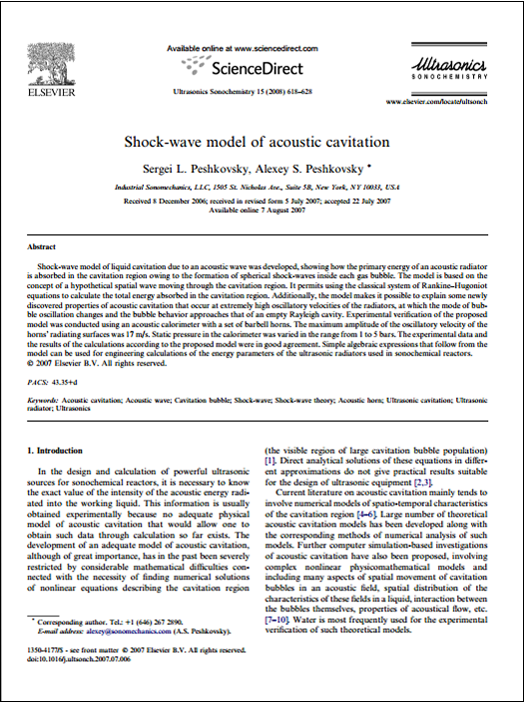# Shock-wave Model of Acoustic Cavitation

• Introduces and explains the shock-wave model of cavitation at low and high acoustic intensities;
• Demonstrates accurate caclulation of the total energy absorbed in the cavitation region, matching experimental results;
• Provides simple equations to determine energy parameters of ultrasonic radiators used in sonochemical reactors.

Sergei L. Peshkovsky, Alexey S. Peshkovsky, Ultrasonics Sonochemistry, Volume 15, Issue 4, April 2008, Pages 618–628.Shock-wave model of liquid cavitation due to an acoustic wave was developed, showing how the primary energy of an acoustic radiator is absorbed in the cavitation region owing to the formation of spherical shock-waves inside each gas bubble. The model is based on the concept of a hypothetical spatial wave moving through the cavitation region. It permits using the classical system of Rankine–Hugoniot equations to calculate the total energy absorbed in the cavitation region. Additionally, the model makes it possible to explain some newly discovered properties of acoustic cavitation that occur at extremely high oscillatory velocities of the radiators, at which the mode of bubble oscillation changes and the bubble behavior approaches that of an empty Rayleigh cavity. Experimental verification of the proposed model was conducted using an acoustic calorimeter with a set of barbell horns. The maximum amplitude of the oscillatory velocity of the horns’ radiating surfaces was 17 m/s. Static pressure in the calorimeter was varied in the range from 1 to 5 bars. The experimental data and the results of the calculations according to the proposed model were in good agreement. Simple algebraic expressions that follow from the model can be used for engineering calculations of the energy parameters of the ultrasonic radiators used in sonochemical reactors.